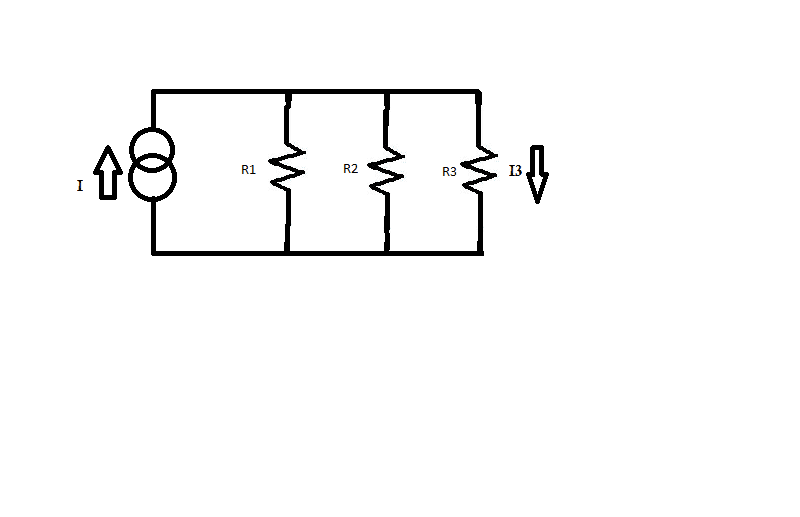# Find the Current Through a Resistor with a Constant Current Source

## Homework Statement

Hey everyone. I might be overthinking this one, but I thought I'd post it. (sorry for the crappy MS Paint sketch!)

I need to find the current through R3## Homework Equations

V=IR
$\Sigma$V=0 (around a closed loop)
$\Sigma$I=0 (going into a junction)

## The Attempt at a Solution

Well my original idea was to write out the value of an equivalent resistor for the 3 resistors in parallel, then use that to find the voltage drop (since it's the same across all 3). However since the values are all different, the algebra got messy pretty quickly, and I thought there would be a much simpler solution to the problem. I'm just a bit thrown off by the constant-current source instead of emf.

Thank you for any and all help!

## Answers and Replies

gneill
Mentor
This is a classic current divider situation. It might be easier to think of it in terms of conductances rather than resistances (G = 1/R). Then the math for the current division looks much like that for a voltage divider.

Write G1, G2, and G3 for the conductances of resistors R1, R2, and R3. Then
$$I_i = I \frac{G_i}{G_1 + G_2 + G_3}$$

Thanks for the quick reply! I see where you're getting at - makes the math much simpler, but we haven't covered conductance yet. Is there any other way around the problem, or do I just have to do some nasty algebra?

gneill
Mentor
Thanks for the quick reply! I see where you're getting at - makes the math much simpler, but we haven't covered conductance yet. Is there any other way around the problem, or do I just have to do some nasty algebra?

It is what it is.... The algebra isn't all that nasty. I've seen much worseStart by writing the equation for the equivalent resistance of all three resistors as you proposed. What is it?

This is a classic current divider situation. It might be easier to think of it in terms of conductances rather than resistances (G = 1/R). Then the math for the current division looks much like that for a voltage divider.

Write G1, G2, and G3 for the conductances of resistors R1, R2, and R3. Then
$$I_i = I \frac{G_i}{G_1 + G_2 + G_3}$$

Well let's see:

$$\frac{1}{R_{eq}} = \frac{1}{R_1} + \frac{1}{R_2} + \frac{1}{R_3}$$

Which I got to:

$$\frac{1}{R_{eq}} = \frac{R_1R_2 + R_1R_3 + R_2R_3}{R_1R_2R_3}$$

Which means:

$$R_{eq} = \frac{R_1R_2R_3}{R_1R_2 + R_1R_3 + R_2R_3}$$

Then I'd plug that in $$V = IR_{eq}$$ and get:

$$V = \frac{IR_1R_2R_3}{R_1R_2 + R_1R_3 + R_2R_3}$$

Then I would just plug that into $$I_3 = \frac{V}{R}$$ and get the answer?

Phew (that equation writing system is crazy!)

Last edited:
gneill
Mentor
Yeah, that's about it.

Note that if you defined $G_i = 1/R_i$ at the outset, your pains would be lessened appreciably:
$$v = I \; R_{eq} = I \frac{1}{G_1 + G_2 + G_3}$$
$$I_3 = \frac{v}{R_3} = v \frac{1}{R_3} = v \; G_3 = I \frac{G_3}{G_1 + G_2 + G_3}$$

Thank you so much for your help! I'm definitely going to look into the Conductance thing there. It looks like a very valuable tool.

gneill
Mentor
Thank you so much for your help! I'm definitely going to look into the Conductance thing there. It looks like a very valuable tool.

You're quite welcome.

Conductances certainly simplify dealing with current dividers. If you must have the results in terms of resistances, you can always substitute them back in for the G's and simplify (sort of like postponing the pain to the end!). If it's a practical circuit you're working on, with actual component values, then you can just use numerical values for the G's and get on with things.